Gage Linearity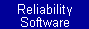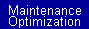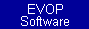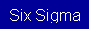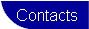Below is a step by step example of how to determine linearity.  The example utilizes the software package Measurement AssuranceClick here to download a free demo version of this software.

To determine linearity, several trials must be made with several standards.  The standards must span the operating range of the gage.  To analyze the data, select Procedures than Linearity from the menu.  The Linearity screen is shown below with sample data.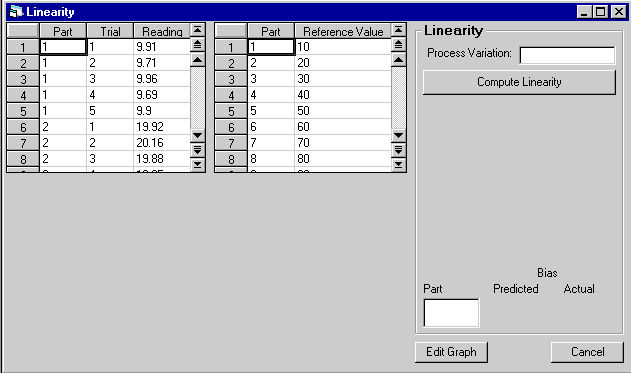When opening and saving files, the files have the extensions "lin" for the readings for each part and trial, and "ref" for the reference values. It is recommended to always use these extensions, because the file extension is used to determine which spreadsheet the file will be loaded into when it is opened. When opening and closing a file, these two file extensions are shown in the "List of files of type:" box. This is shown below.Click the "Compute Linearity" button to generate the results of the study.  The "Linearity" section displays the output for the linearity procedure. The "process variation" is not required input. If the process variation is not input, linearity will only be computed as a percent. This section also gives the equation of the best fit line in the linearity plot, the "Goodness of Fit", which is equal to the coefficient of determination of this line, and a spreadsheet that displays the calculated bias for each reference value. The predicted bias and actual bias for any of the reference values can be computed by selecting the desired reference part in the "Part" box at the bottom of the "Linearity" section.  This is shown below.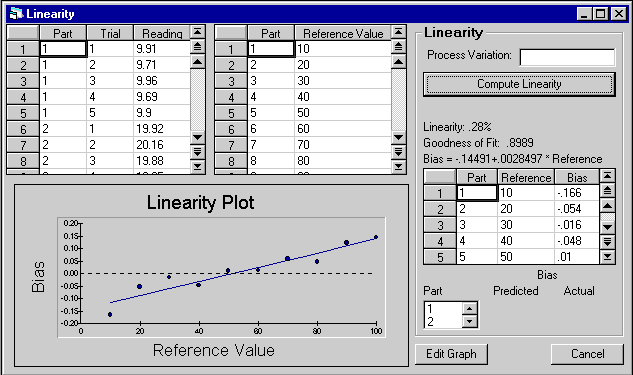Engineered Software, Inc. 734-502-8582 Support@EngineeredSoftware.com